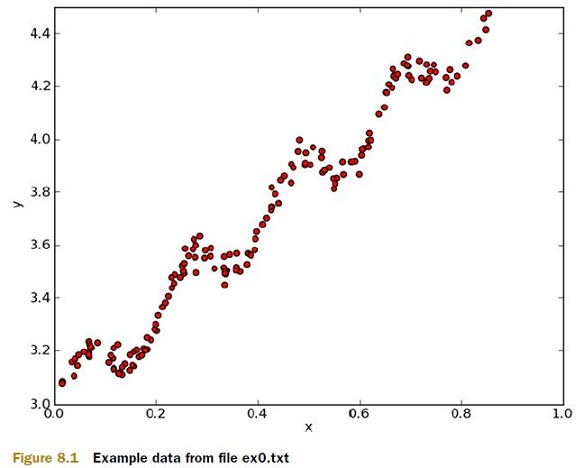## 2012年9月3日 星期一

### [ ML In Action ] Predicting numeric values : regression - Linear regression (1)

Preface :

Finding best-fit lines with linear regression :

Linear regression
Pros: Easy to interpret result, computationally inexpensive
Cons: Poorly models nonlinear data
Works with: Numeric values, nominal values

HorsePower = 0.0015 * annualSalary - 0.99 * hoursListeningToPublicRadio

General approach to regression
1. Collect: Any metod
2. Prepare: We'll need numeric values for regression.
3. Analyze: It’s helpful to visualized 2D plots. Also, we can visualize the regression weights if we apply shrinkage methods.
4. Train: Find the regression weights.
5. Test: We can measure the R2, or correlation of the predicted value and data, to measure the success of our models.
6. Use: With regression, we can forecast a numeric value for a number of inputs. This is an improvement over classification because we’re predicting a continuous value rather than a discrete category.1. #!/usr/local/bin/python
2. # -*- coding: utf-8 -*-
3. from numpy import *
4.
6.     """ General function to parse tab -delimited floats. """
7.     numFeat = len(open(fileName).readline().split('\t')) - 1 #get number of fields
8.     dataMat = []; labelMat = []
9.     fr = open(fileName)
11.         lineArr =[]
12.         curLine = line.strip().split('\t')
13.         for i in range(numFeat):
14.             lineArr.append(float(curLine[i]))
15.         dataMat.append(lineArr)
16.         labelMat.append(float(curLine[-1]))
17.     return dataMat,labelMat

>>> import regression
>>> from numpy import *
>>> xArr[0:2]
[[1.0, 0.067732000000000001], [1.0, 0.42781000000000002]]

1. def standRegres(xArr,yArr):
2.     xMat = mat(xArr); yMat = mat(yArr).T
3.     xTx = xMat.T*xMat
4.     if linalg.det(xTx) == 0.0:
5.         print "This matrix is singular, cannot do inverse"
6.         return
7.     ws = xTx.I * (xMat.T*yMat)
8.     return ws

>>> ws = regression.standRegres(xArr, yArr)
>>> ws
matrix([[ 3.00774324],
[ 1.69532264]])

Y = 1.69532264X + 3.00774324

>>> xMat = mat(xArr)
>>> yMat = mat(yArr)
>>> yHat = xMat * ws # yi = xi*w
>>> import matplotlib.pyplot as plt
>>> fig = plt.figure()
>>> ax.scatter(xMat[:,1].flatten().A, yMat.T[:,0].flatten().A)

>>> xCopy = xMat.copy()
>>> xCopy.sort(0)
>>> yHat = xCopy * ws
>>> ax.plot(xCopy[:,1], yHat)
[]
>>> plt.show()Supplement :
[ ML In Action ] Predicting numeric values : regression - Linear regression (1)
[ ML In Action ] Predicting numeric values : regression - Linear regression (2)
[ ML In Action ] Predicting numeric values : regression - Linear regression (3)

### [ Python 文章收集 ] Monitoring memory usage of a running Python program

Source From  Here Preface At  Survata , we do a lot of data processing using Python and its suite of data processing libraries like  pandas ...